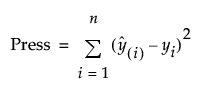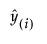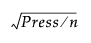Publication date: 11/10/2021

## Press

The Press, or prediction error sum of squares, statistic is an estimate of prediction error computed using leave-one-out cross validation. In leave-one-out cross validation, each observation, in turn, is removed. Consider a specific observation. The model is fit with that observation withheld and then a predicted value is obtained for that observation. The residual for that observation is computed. This procedure is applied to all observations and the residuals are squared and summed to give the Press value.

Specifically, the Press statistic is given by the following:where n is the number of observations, yi is the observed response value for the ith observation, andis the predicted response value for the ith observation. These values are based on a model fit without including that observation.

The Press RMSE is defined as.

The Press RSquare is defined as 1 - Press/SSTotal.